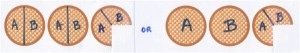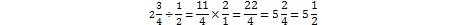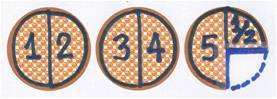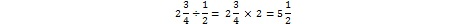Recent Posts
Moving From Product to Process Success Criteria
Connect with:
Saturday / June 15

Rethinking Fraction Division: One of the Hardest Topics to Teach and LearnThe old adage for dividing fractions, “Ours is not to reason why, just invert and multiply!” is one many readers are certainly familiar with. And because students often forget which fraction to invert, other less known adages have arisen lately. I learned this one last year from a 4th grade teacher: “Flip the fellow that follows.”While students should know their procedures, I think we all would agree that students should develop conceptual understanding in tandem with procedural fluency (NRC, 2001). Especially in a technological world, in which young and old have a plethora of computational tools are at their fingertips, students should be able to make sense of procedures they use, understand why they work, and have a repertoire of mental images that help them make sense of them (see my post, “How Can We Plant the Seeds of Algebra in the Elementary Grades?”).

If you are wondering, “Well, why do we invert and then multiply?” or if you are having a hard time to come up with a situation illustrating fraction division in a way that’s meaningful to a 4th, 5th, or even a 6th grader, rest assured you are not alone (Borko et al., 1992; Philipp, 2000): fraction division is probably the hardest elementary topic to teach. Recently, a seasoned professional development provider was unable to come up with a real world example for a group of upper elementary teachers.

The reasons why students (and sometimes teachers) have difficulties with fraction division are many, most of which I can’t expand on in a blog post (for detailed information, see my book, Planting the Seeds of Algebra, 3-5, coming out this fall). However, I name some here and then develop two below. Understanding multiple meanings of the symbol a/b, the importance of the unit, multiplicative thinking, measurement division, the connection between division of whole numbers and division of rational numbers, and the inverse relationship between multiplication and division are all necessary for fraction division competence, both procedural and conceptual.

Example 1: “What is 1/3 of 1/2?”

A few months ago in a 6th-grade classroom of an international school I asked, “What is 1/3 of 1/2?” A girl answered, “My teacher taught me last year that ‘of’ means times, so it’s 1/3 x 1/2 = 1/6.” I acquiesced and pushed further: “Using manipulatives or telling a story, can you give an example that would help a younger student make sense of this problem?” Silence. Alina volunteered, “I don’t know why we get 1/6; I just remember that ‘of’ means ‘times’ and I do it.”

I continued. “Let’s go back to whole numbers for a moment; what would 1/3 of 15 be?” Instantly, Alina replied, “Five.” When asked what reasoning led to her answer, she replied, “I split 15 in three parts in my head and I know it’s 5.” She saw clearly that a third of a whole fits three times inside the whole. Capitalizing on her understanding, I drew a rectangle on the board and shaded in one half (Figure 1). “Using your reasoning, can you draw 1/3 of the blue area?” I nudged further. Reacting to the task with, “That’s easy,” Alina simply divided the blue rectangle into three equal parts and shaded one of them in black. Seconds later, with a Eureka grin on her face, she exclaimed, “Oh! That’s one sixth!”Lesson One: Memorizing Sometimes Contradicts Sense Making

I ask this question a lot and watch carefully what students do. Often, they draw a circle, shade in a half, and proceed to divide the half into three parts to get one sixth, just as Alina ultimately did. And then I comment, “Hmm, you said ‘of means times’ yet you are dividing; how come?” They’re confused. Indeed, when students take (1/d)th of a quantity or area, they divide the quantity or area into d equal parts and then shade one part. Their instinctive action is therefore division yet we tell them that “of means multiplication.” Confused, they quickly surrender their sense making to rote memorization.

Lesson Two: Connecting Multiplication and Division as Inverse Operations

Is Example 1 a fraction division problem or a fraction multiplication problem? Good question, right? The action of dividing the half into three equal partsto get 1/6 is precisely what confuses many children and adults into thinking that this is a fraction division problem. [Another fact that leads students to think “division” is the resulting, smaller fraction of 1/6: students incorrectly apply to rational numbers the rule “division makes smaller,” which they discover while working with whole numbers.] A mathematical way of reconciling the multiplication of fractions with the action of division, is to emphasize that:

• Multiplying by 1/2 is equivalent to dividing by 2/1 or 2;
• Multiplying by 1/3 is equivalent to dividing by 3/1 or 3;
• Multiplying by 1/4 is equivalent to dividing by 4/1 or 4; or in general

Multiplying by 1/n is equivalent to dividing by n/1 or n.

This not only conveys the meaning of the denominator of a rational number, a/b, as a divisor, but also underlines the inverse relationship between multiplication and division, which students are told—and they repeat—but with few illustrations. Over time, this inverse relationship generalizes to any rational number a/b:

• Multiplying by a/b is equivalent to dividing by b/a.

Example 2: “Give meaning to 2¾ ÷ ½ with a story and without the algorithm.”This is another one of my favorite questions. I pose it to parents at Math Nights and to teachers at PD sessions. Invariably, I get a story about two-and-three-quarters pizzas divided between two people. So I oblige my audience and draw the picture, labeling the two people A and B (Figure 2). A majority agrees without computation that each share is approximately one-and-a-third-pizza, and is ready to move on. But (thankfully) there is always someone who can’t resist the urge to apply the division algorithm. I spot the disobeyer shaking his or her head in disagreement because the result of “flip the fellow that follows” gives,“We now have a dilemma,” I comment: “A moment ago, we were convinced that person A and person B each gets and equal share of less than two pizzas; now we’re told the correct answer, produced by the algorithm, is five-and-a-half! Can anyone make sense of this apparent contradiction?” Dead silence…

Lesson Three: Two Interpretations of Division

The tendency of students and adults to want to “divide between two people” is due to the predominance of “fair-share” or partitive division examples, versus the sparseness of “measurement” or quotative division examples, we model for young children when first introducing division with whole numbers. To rectify this, the Common Core Standards urge us to model both interpretations of division beginning in Grade 3. Standard 3.OA.A.2 clearly states,

“Interpret 56 ÷ 8 as the number of objects in each share when 56 objects are partitioned equally into 8 shares, or as a number of shares when 56 objects are partitioned into equal shares of 8 objects each” (the first being partitive division and the second quotative).

Lesson Four: Model Multiple Contexts of Fraction Division for Students

Quotative division comes to our rescue. I stay with the two-and-three-quarters pizzas but tell a different story: Mr. Cardone is the owner of a Manhattan pizza carryout. Since 50% of Manhattan residents live alone, his unit portions are half-pizzas. He closes shop at 9:00 p.m. At 8:00 p.m., there are two-and-three-quarters pizzas left. Help Mr. Cardone figure out how many portions he can make from the remaining pizzas? The answer is clearly 5 ½ (Figure 3), and it can be obtained without forfeiting sense making or logical reasoning. Since students need multiple contexts of any concept we teach in order to deepen their understanding of it, see if you can come up with other stories for “÷ ½.” Let’s all list them at the bottom of this blog post, thus providing help to many teachers to teach the hardest topic in elementary math, and students to learn it with understanding! [I offer other examples in my upcoming Corwin Press book.]Epilogue

Two points:

1. Applying the rule stated above, namely, “Multiplying by a/b is equivalent to dividing by b/a” explains the adage “invert and multiply” in a mathematical way. So dividing by ½ is like multiplying by 2:2. Note that the unit of the quotient is not 5½ pizzas but rather 5½ half-pizzas—thus the importance of the unit when working with fractions!